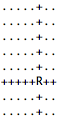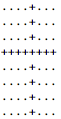## Saturday, November 24, 2012

### Chess in C (Part 3) - Rook, Rooks, Rookies, Wookies, same thing

Today's blog post will be short like the sentences of a language I'm learning. ¿Comprendes? I'm not learning Spanish, I've just wanted to use one of those upside down question marks. Twenty years I've been waiting to use those question marks.

I'll cover one chess piece, and one chess piece only! I'm assuming you've read Chess in C parts one and two. In part one, we covered the chess board and how to represent pieces. In part two, we covered how to model moves of pawns as well as a general approach to calculating whether a square is a potential destination for piece. Before we begin today, grab the code at the end of part two and compile it. We're going to make some modifications to this code, and I'm assuming you're ready for some hacking.

Today's piece is a rook. In chess, a rook is a piece that can move up, down, left or right any number of squares. Here's what a rook looks like.It's also the logo of about 642 financial services companies worldwide.

And here are the potential moves of a rook. We're going to write code to calculate the potential moves.The rook is in (3, 4). Remember, we start counting at 0. And it's not a regular Cartesian plane.

The castle represents the rook and the X represents the potential moves. The diagram assumes there aren't any obstructions in the way like other pieces or a banana peel. So, for any given square, how can we calculate whether there is it is a possible move for a rook?

Here's the aid again. Each cell is represented by a pair of integers (X and Y). X represents row and Y represents column. The first square (0,0) is in the top-left corner. Don't get it confused with a Cartesian plane (the ones you probably learnt in math class) where (0,0) is in the bottom-left corner.Made in Microsoft Word.

In our example, the rook is in (3, 4). That means

1. every square on that row (row 3) is a possible move.
2. every square in that column (column 4) is a possible move.
Now, get some paper and write every potential move if the rook is in (3,4). Come on! It may seem obvious, but you need realise that something will be missing. STOP READING THIS BLOG POST AND ACTUALLY WRITE THEM DOWN!

You done? Alright, you should have written down coordinates covered by the Xs. Let's check.

Maybe you started writing down the columns, maybe the rows. Doesn't matter. You should have written down the following:

Squares in column 4:
(0,4), (1,4), (2,4),        (4,4), (5,4), (6,4), (7,4)

Squares in row 3:
(3,0), (3,1), (3,2), (3,3),        (3,5), (3,6), (3,7)

If you wrote down these coordinates, congratulations! If you wrote down (3,4), then you're wrong! Square (3,4) isn't a potential move because that's where the rook is! As I said, it seems obvious. But keep it in mind when we write some conditional (if) statements. That's what I wanted you to realise: we're going to need to come up with a way to ignore the current square

I'm going to grab the code for iterating through the chess board from part one and modify it a little. You should understand this code and be able to write it without stressing.

For the purposes of demonstration, I'm going to make two variables that contain the row and column of a rook. This isn't how you'd store the location of a rook: it's just a quick way of showing you how to detect a rook in the current row or column.

This consists of two compound if statements within the nested for loop.

The first if statement checks the row for the rook, and the second if statement checks the column for a rook. The first if statement tests for two conditions:

1. Whether the current row (iterated through by variable i) is equal to the row that contains the rook (pieceRow). If this statement is true, then every square is marked as a potential move EXCEPT if the square is in the column containing the rook (pieceColumn). If it didn't check this, every square in the row would be a potential move (even the piece containing the rook!). We know this isn't true from our exercise above: the piece cannot move into its own square.
2. Whether the current column (iterated through by variable j) is equal to the column that contains the rook (pieceColumn). If this statement is true, then every square is marked as potential move EXCEPT if the square is in the row containing the rook (pieceRow). If it didn't check this, every square in the column would be a potential move (even the piece containing the rook!) We know this isn't true from our exercise above: the piece cannot move into its own square.
If you're confused, the best way to understand is to write the code and see what happens, then break the code and see what happens. In fact, let's do this. Copy and paste this code.

Now compile it. If all went to plan, your board should look like this.

Lookin' good! (rook is in (3,4) - remember, start counting from 0)

Let's test a few more possibilities! Replace the pieceRow and pieceColumn with 5 and 5. You should get this.Also lookin' good! (rook is in (5,5) - remember, start counting from 0)

You might also want to test some edge cases like (0,0) and (7,7) to make sure the algorithm works.

Now, let's break this thing! If you haven't broken anything while coding, make sure you're actually coding because making mistakes is part of the process. Remember before how I said we had to make sure not mark the rook's current position as a possible move? We tested for that condition by putting a != pieceColumn and != rowColumn in the appropriate if statement. Let's remove that and see what happens!The rook no longer exists. It has been clobbered!
So, I think we have the code nailed down for calculating possible rook moves. Of course, it's not in a usable form. You have to manually put the position of the rook into pieceRow and pieceColumn. So I'm going to set some homework for you: try and modify the code so that when you specify a rook in the initial start position, the code will pick that up and automatically draw it. I'll write the answer in my next blog post (this year, I promise!)

Other posts in this series

Chess in C (Part 1)
Chess in C (Part 2) - Insert Pawn Pun Here
Chess in C (Part 3) - Rook, Rooks, Rookies, Wookies, same thing
Chess in C (Part 4) - I'm asking for input
Chess in C (Part 5) - Potential moves of a bishop: up-left, cardinal, pope

#### 1 comment:

1.Hi
Thanks for part3. This code works but I am playing around with it. I have uncommented all pieces/pawns so they appear in their start positions & added the calculate pawn move code from Part2 to this so I see the possible pawn moves but it does not show the potential rook moves? It's no big deal for now & I look forward to more posts and getting toward some useable console chess game in C with this?! Also so far I have failed on the homework-I'm an F grade student... Thanks :-)